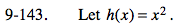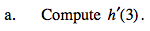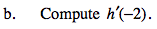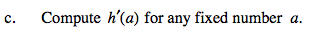### Home > PCT > Chapter Ch9 > Lesson 9.3.4 > Problem9-143

9-143.Find the general slope function at any x.

$\lim_{h\rightarrow 0}\frac{h(x+h)-h(x)}{x+h-x}=\lim_{h\rightarrow 0}\frac{(x+h)^{2}-x^{2}}{h}=\lim_{h\rightarrow 0}\frac{x^{2}+2xh+h^{2}-x^{2}}{h}=\lim_{h\rightarrow 0}\frac{2xh+h^{2}}{h}=\lim_{h\rightarrow 0}\frac{h(2x+h)}{h}=\lim_{h\to 0}2x+h=2x$Substitute the input value in the general slope function.−42a